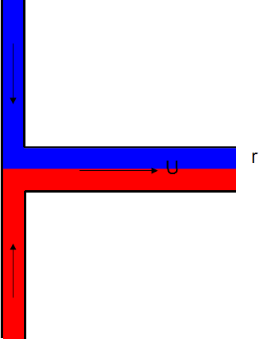## T-Mixer

### Quick Start

You might think that it would be easy to mix two capillary flows by just bringing them together. Yet it takes a surprisingly long time, forcing the need for complicated mixing geometries.

The two key parameters are Radius and Diffusion coefficient. The time and length for mixing are calculated. Your actual Length tells you whether you can mix OK and when combined with Viscosity it gives you the pressure needed to generate the flow.

### T-Mixer

D *10-7 cm²/s
Length L mm
Viscosity η cP
Speed U mm/s
tmix
Lmix
Q
PQMixing two liquids in capillaries seems easy: bring them together at a T-junction and let diffusion do the work. The size of the capillaries is so small that surely diffusion will be very fast. Sadly this isn't the case. In many/most cases, T-junction mixers are useless. The time taken to mix in a capillary of radius r and with a diffusion coefficient, D is given by:

t≃r^2/D

If the flow velocity is U then the length, L, needed to fully mix is given by:

L≃(Ur^2)/D

The pressure needed to provide the flux Q=Uπr² with flow of velocity U is given by Poiseuille as:

P_Q=(8QLμ)/(πr^4)=(8ULμ)/r^2

The calculation provides the numbers and the colour of the Lmix box warns (pink) if the mixing length is greater than the actual length L.

What are typical values of D? For small molecules it is something like 2e-5cm²/s (a value of 200 in the slider) and for molecules such a proteins it might be 2e-7cm²/s (a value of 2 in the slider.

What the app shows is that for "large" radius capillaries the mixing length is hopelessly large unless the flow rate is very low. For "small" capillaries the mixing length is reasonable, but the liquid throughput is very small, OK for diagnostic tests but useless for any sort of flow reactor. This means that any realistic type of mixer should use other tricks such as adding obstructions and bends to create turbulence, to flow at a higher (turbulent) Reynolds number or create specific structures to produce sophisticated flows such as chaotic advection.